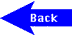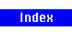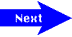# National Aeronautics and Space Administration

## Goddard Space Flight Center## Life Cycles of Stars (Grades 9-12) - Page 15

1. Solve Wien's Law for T, substitute in the values for wavelength. With the temperature you obtain, look on the H-R diagram for the corresponding spectral class.

(a) 9656 K Class A; (b) 19,313 K Class B; (c) 5267.2 K Class G; (d) 2317 K Class M

2. Substitute the temperatures into Wien's Law and obtain the wavelengths of the peak emission. Look up on a chart of the EM spectrum which region the wavelength falls into.

(a) 289.7 cm radio; (b) 3.62x10-4 cm infrared; (c) 1.93x10-5 cm ultraviolet;

(d) 1.65x10-7 cm X-ray

Extension:

No astronomical objects are as cold as 0.001 Kelvin. The radio emission we observe is produced by electrons moving in magnetic fields (this is called synchrotron radiation).

Using the equation: distance = velocity x time,

Cygnus: 9.14x1014 km; Crab: 4.46x1013 km; Tycho: 6.96x1013 km; SN1006: 9.37x1013 km

The supernova occurred in the year 1604 and is known as Kepler's supernova. It was observed and documented by the astronomer Johannes Kepler.

A Teaspoonful of Starstuff

Using the equation: mass = density x volume,

We are given that the volume of interest is 5 cm3. So what is the density of each of the objects? Density equals mass/volume, and the volume of a sphere is 4/3 πr3, where r is the radius of the sphere. Plugging in the values for each of the types of stars, we find that our teaspoon of the Sun would contain 7.0 grams; of the white dwarf would contain 9.5x106 grams; of the neutron star would contain 3.3x1015 grams. By looking up the density of water, air, and iron, you can calculate that each would be 5.0 grams, 6.5x10-3 grams, and 39.4 grams, respectively.

Crossing the Event Horizon

1. Using the Schwarzschild equation, we input the mass of Jupiter (1.9x1027 kg), the Gravitational constant (G = 6.67x10-11 m3/kg-sec) and the velocity of light (3x108 m/sec) to see that the event horizon of a Jupiter-mass black hole would occur at 2.96 meters.